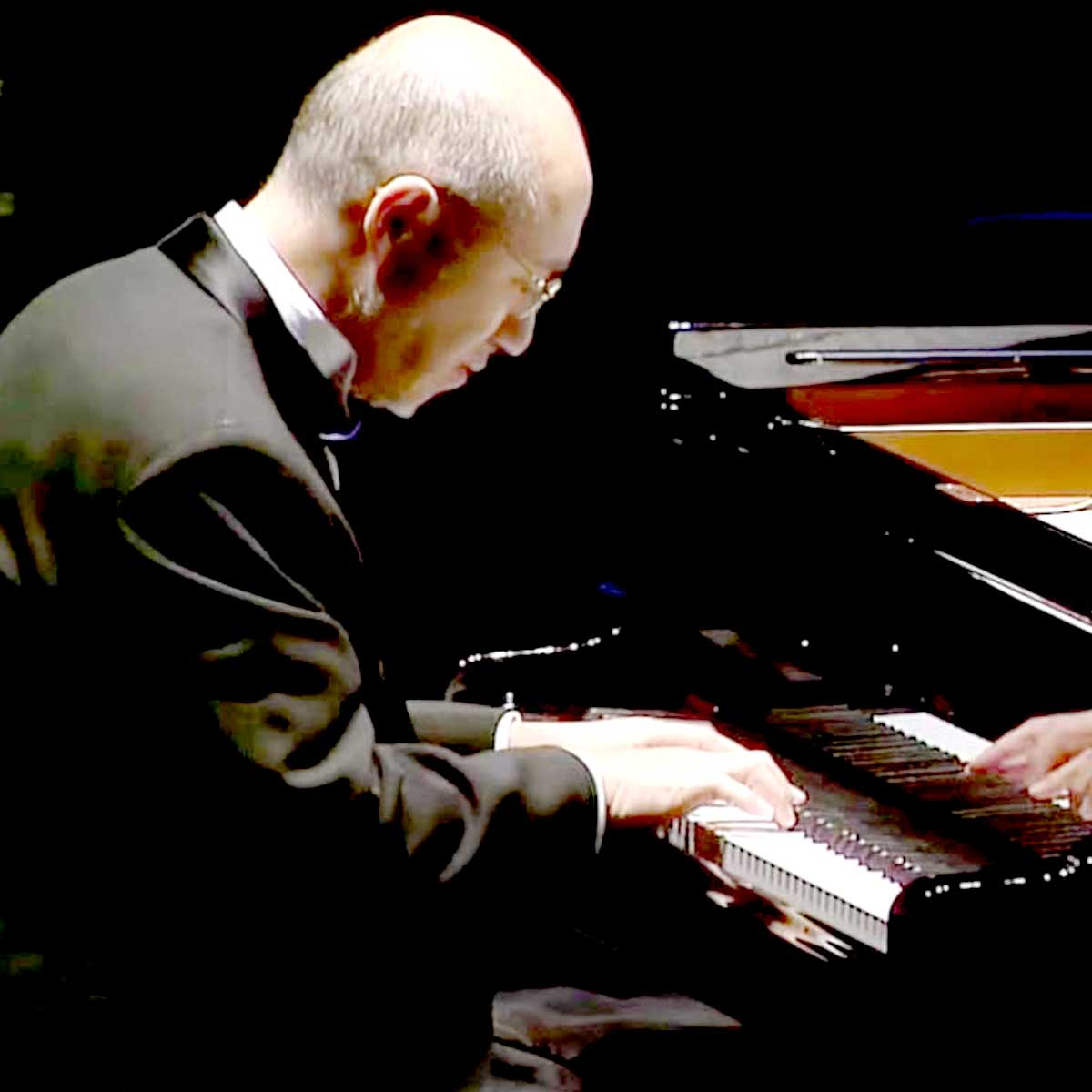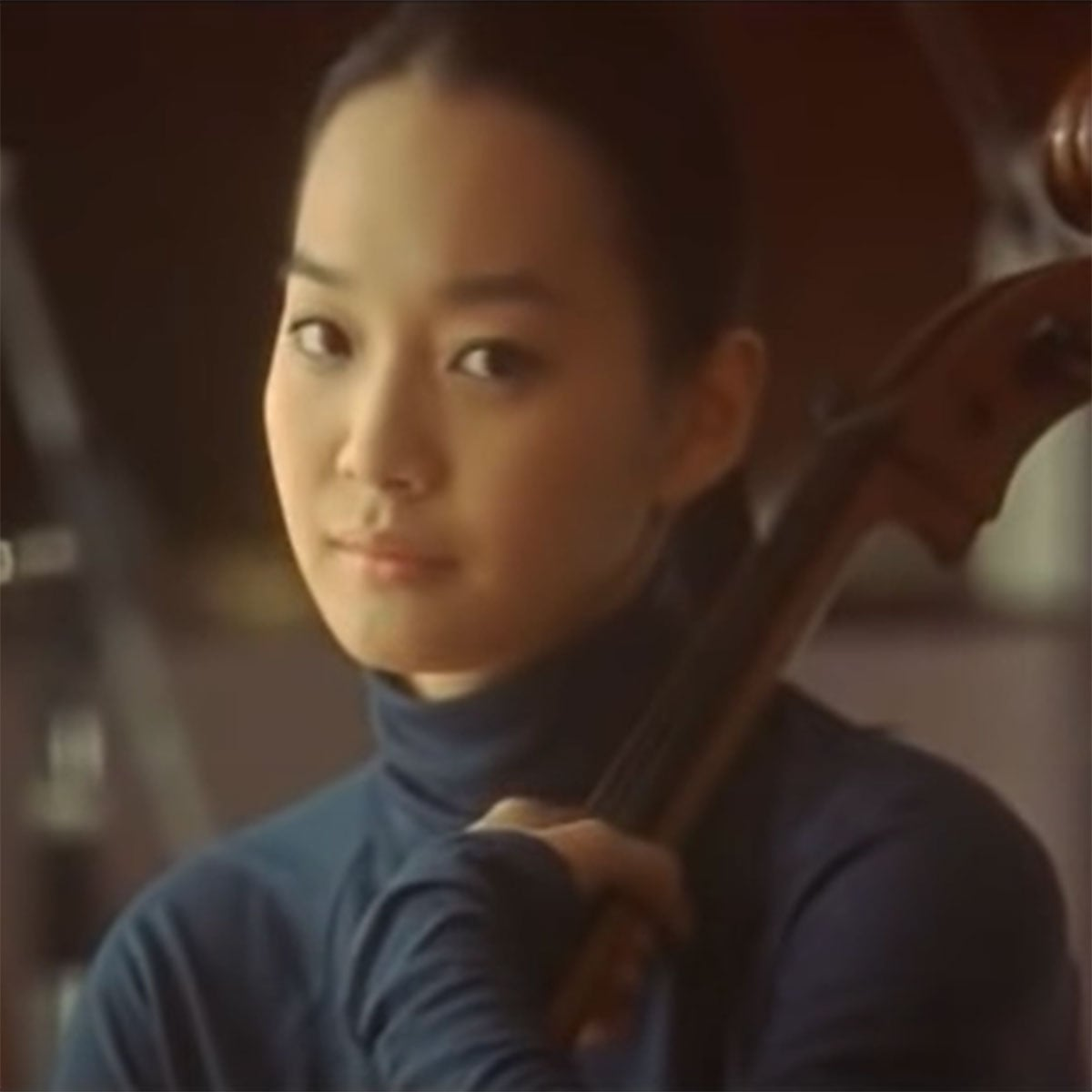# Yuhki Kuramoto

1 Music Sheets

Yuhki

• ###### Born:

10th September 1951

Yuhki Kuramoto (倉本 裕基, Kuramoto Yūki, born September 10, 1951) is a Japanese pianist and composer. His given name is Minoru Kitano (北野 實, Kitano Minoru). He writes primarily for the piano, though orchestral arrangements for some of his pieces exist. Born in Urawa-ku, Saitama, Kuramoto studied the piano from an early age. At school, he studied Rachmaninoff and performed as a part-time soloist in orchestras. He later went on to study at the Tokyo Institute of Technology and obtained a master's degree in Applied Physics. Kuramoto chose to become a musician. Kuramoto concentrated on the performance of the piano, the composition and arrangement of classical music and popular music. As a professional musician, his interests span genres from classical to folk songs and popular music. Kuramoto never attended a music conservatory and attained his knowledge mostly through self-study.

## Artist's Music Sheets

•[jQ] [tG] [sI] p [h0] [rf] [ua] o [QD] [ea] [pY] I [wf] [ra] u r 6 [f0] [rd] [ts] [sW] e 0 [d8] [a7] Q [reY] w Q e [rY] I [a0] o [uf] r [G9] a [uh] r [k] e [uj] e u p f p [h8] e [upG] e 7 [fe] [pID] G [f0] r [uo] [ra] [aYI] r [uo] r [p8] u [tf] e [D7] e [rpG] Y [f0] r [uoa] [YI] [uo] a [urp] o[s6] 0 [ute] w Q e [ut] d [a7] Q [reY] w Q e [rY] a [a0] o [uf] r [G9] a [uh] r [k] e [uj] e u p f p [h8] e [upG] e 7 [fe] [pID] G [f0] r [uo] [ra] [aYI] r [uo] r [p8] u [tf] e [D7] Q [rG] 6 [f5] r [wuk] r [l6] t [jie] t [h7] r u o 7 [eG] [rf] ID[f0] w r u Y u r w [x6] 0 t [yf] [uf] t o t [I9] j k l [utkQ] j H j [z5] 9 r [dT] [yd] r I r [wu8] h j k [ywj0] h G h [l\$] 8 e [sr] [ts] e u e [Y7] k j H [rjI] D f G [h0] r u r [jQ] r Y r [wk] r u r W [rk] [yl] [zW] [xl6] 0 t [yf] [uf] t [so] t [aI9] x z l [tpkI] j k l [z7] Q r [dT] [yd] r [aI] r [ypI0] z l k [yuroj] h j k [l6] 0 t [ys] [us5] t u p [u\$]psfk j H [tje] s h G [f7] e r t D r a r [h7] T P T [G7] Y p Y [a0] o [uf] r [G9] a [uh] r [k] e [uj] e u p f p [h8] e [upG] e 7 [fe] [pID] G [f0] r [uo] [ra] [aYI] r [uo] r [p8] u [tf] e [D7] e [rpG] Y [f0] r [uoa] [YI] [uo] a [urp] o[s6] 0 [ute] w Q e [ut] d [a7] Q [reY] w Q e [rY] a [a0] o [uf] r [G9] a [uh] r [k] e [uj] e u p f p [h8] e [upG] e 7 [fe] [pID] G [f0] r [uo] [ra] [aYI] r [uo] r [p8] u [tf] e [D7] Q [rG] 6 [f5] r [wuk] r [l6] t [jie] t [h7] r u o 7 [eG] [rf] ID[f0] w r u Y u r w 3 7 0 w Q w 0 7 [x6] 0 t [yf] [uf] t [so] t [aI9] j k l [tpkI] j H j [z5] 9 r [dT] [yd] r [aI] [yr] [pI0] h j k [yuroj] h G h [l\$] 8 e [sr] [ts] e [up] e [oY7] k j H [jeYI] D f G [h0] r u r [jQ] r Y r [wk] r u r W [rk] [yl] [zW] [xl6] 0 t [yf] [uf] t [sof] t [daI9] x z l [yspkI] j k l [z7] Q r [dT] [yd] r [daI] r [spI0] z l k [uoja] h j k [l6] 0 t [ys] [us5] t u p [u\$]psfk j H [tje] s h G [f7] e r t D r a r [h7] T P T [G7] Y p Y [a0] o [uf] r [G9] a [uh] r [k] e [uj] e u p f p [h8] e [upG] e 7 [fe] [pID] G [f0] r [uo] [ra] [aYI] r [uo] r [p8] u [tf] e [D7] e [rpG] Y [f0] r [uoa] [YI] [uo] a [urp] o[s6] 0 [ute] w Q e [ut] d [a7] Q [reY] w Q e [rY] a [a0] o [uf] r [G9] a [uh] r [k] e [uj] e u p f p [h8] e [upG] e 7 [fe] [pID] G [f0] r [uo] [ra] [aYI] r [uo] r [p8] u [tf] e [D7] Q [rG] 6 [f5] r [wuk] r [l6] t [jie] t [h7] r u o 7 [eG] [rf] ID[f0] w r u Y u r w 6 0 [urf]p t [dW] e [s0] 6 [a7] [oe] [rI] [ut] [rY]|[uaI]|[uoa3] 7 0 w r w r u [f0][wh][rk]x

Level: 6
Length: 03:47
Intermediate